Question

# How much 12 M HCI would you need to make 100.0 ml of 2.0 M HCI?

How much 12 M HCI would you need to make 100.0 ml of 2.0 M HCI?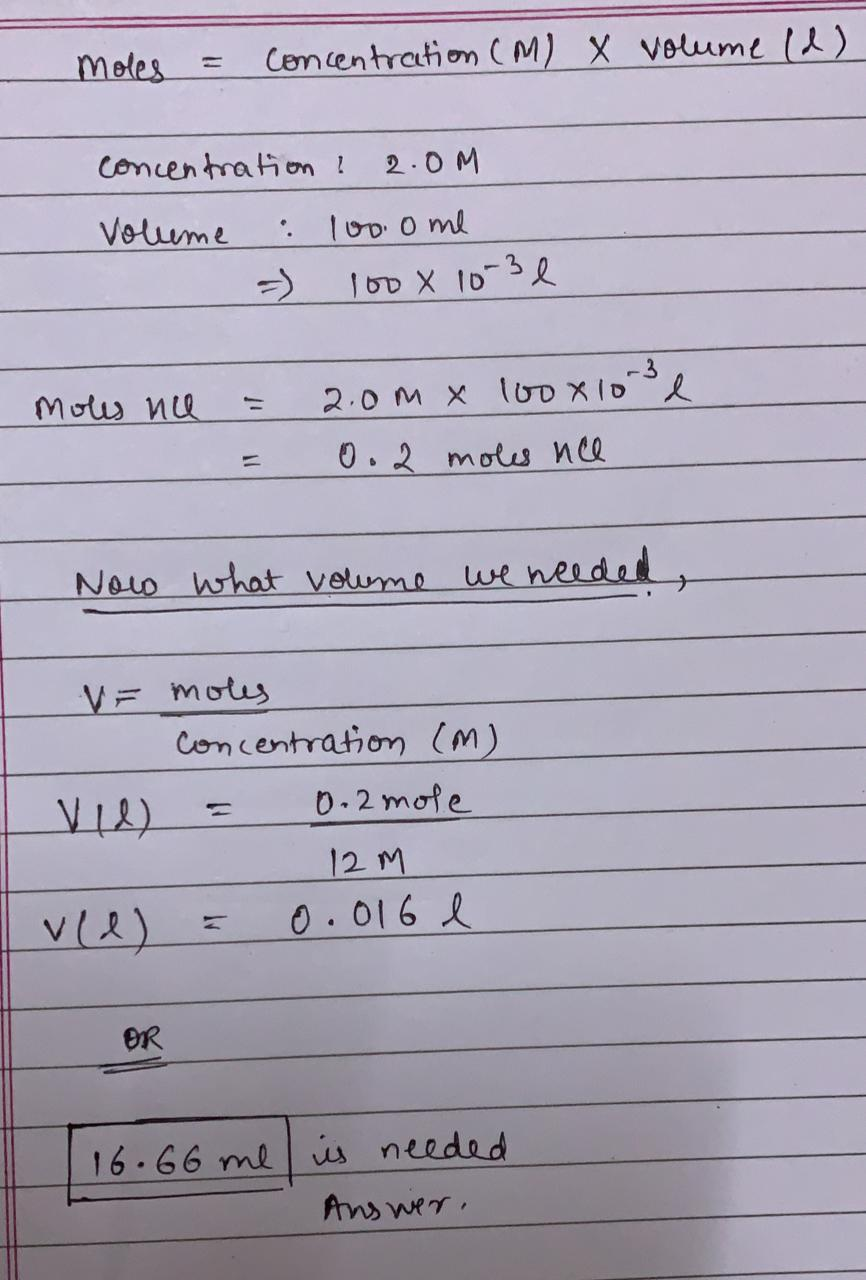#### Earn Coins

Coins can be redeemed for fabulous gifts.

Similar Homework Help Questions
• ### Part A Describe how you would make 100.0 mL of a 1.00 M NaOH solution from...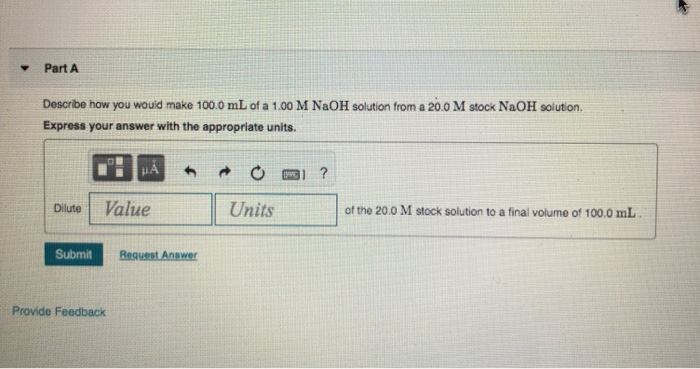Part A Describe how you would make 100.0 mL of a 1.00 M NaOH solution from a 20.0 M stock NaOH solution. Express your answer with the appropriate units. L! - m o mi? Dilute Value Units of the 20.0 M stock solution to a final volume of 100.0 mL Submit Request Answer Provide Feedback

• ### How much water would you need to add to 10.0 mL of 0.500 M HCl to...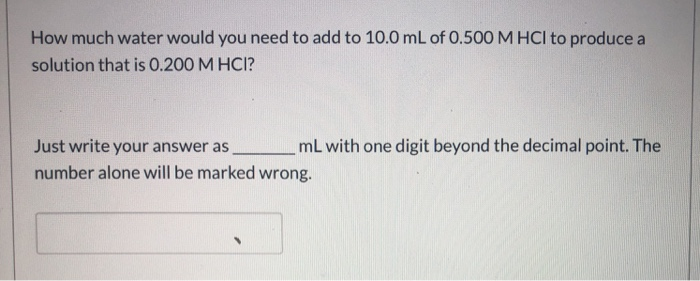How much water would you need to add to 10.0 mL of 0.500 M HCl to produce a solution that is 0.200 M HCI? Just write your answer as mL with one digit beyond the decimal point. The number alone will be marked wrong.

• ### how many milliliters of a stock solution of 2.00 M KNO3 would you need to prepare 100.0 mL of 0.150 M KNO3

how many milliliters of a stock solution of 2.00 M KNO3 would you need to prepare 100.0 mL of 0.150 M KNO3?

• ### You want to make 850.0 mL of a 2.0 M solution of MgBr2. a. How many...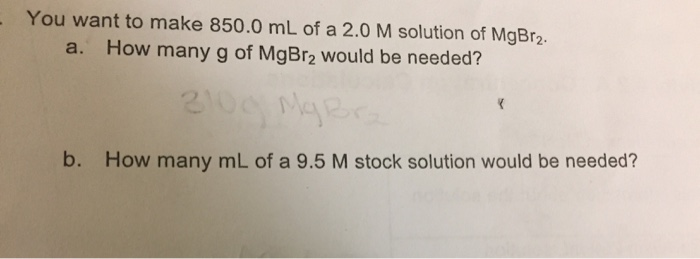You want to make 850.0 mL of a 2.0 M solution of MgBr2. a. How many g of MgBrą would be needed? b. How many mL of a 9.5 M stock solution would be needed?

• ### You need to prepare 100.0 mL of a pH=4.00 buffer solution using 0.100 M benzoic acid...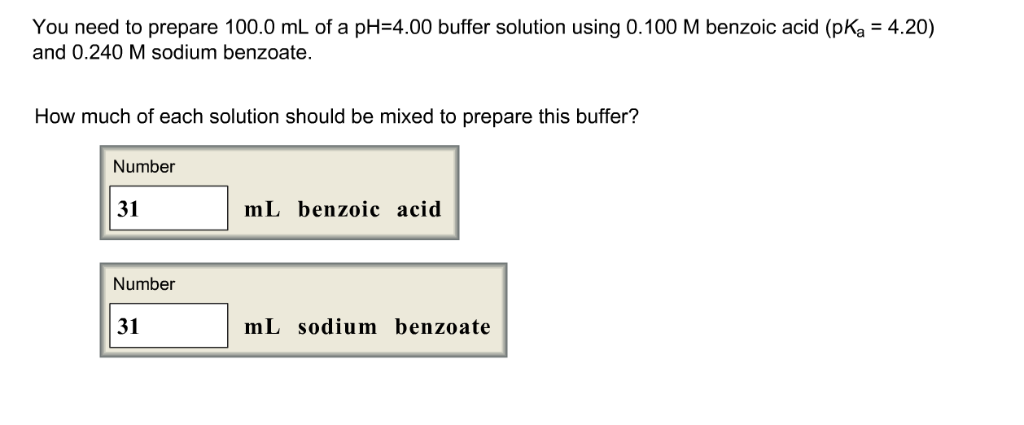You need to prepare 100.0 mL of a pH=4.00 buffer solution using 0.100 M benzoic acid (pKa = 4.20) and 0.240 M sodium benzoate. How much of each solution should be mixed to prepare this buffer? You need to prepare 100.0 mL of a pH-4.00 buffer solution using 0.100 M benzoic acid (pKa 4.20) and 0.240 M sodium benzoate. How much of each solution should be mixed to prepare this buffer? Number 31 mL benzoic acid Number 31 mL sodium...

• ### If I mixed 100.0 mL of a nitric acid solution of pH = 2.40 with 100.0...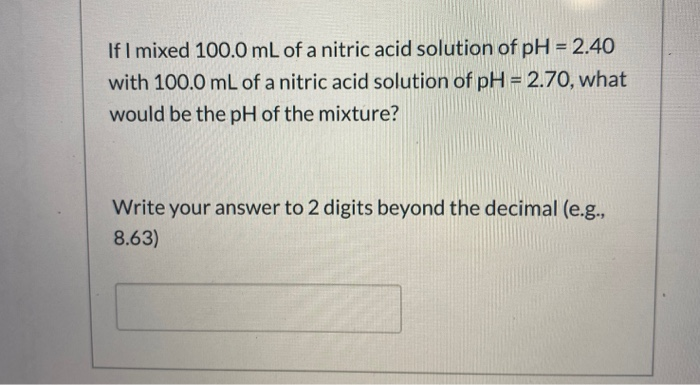If I mixed 100.0 mL of a nitric acid solution of pH = 2.40 with 100.0 mL of a nitric acid solution of pH = 2.70, what would be the pH of the mixture? Write your answer to 2 digits beyond the decimal (e.g., 8.63) If 5.15 grams of iron(III) nitrate (molar mass = 241.86 g/mol) is dissolved in enough water to prepare exactly 150.0 mL of solution, what would be the molar concentration of the nitrate ion? 0.284 M...

• ### Which of the following could you add to 100.0 mL of 1.0 M HCN to make...

Which of the following could you add to 100.0 mL of 1.0 M HCN to make a suitable buffer system? (There may be more than one correct answer.) 100.0 mL of 0.050 M NaCN, 100.0 mL of 0.20 M NaCN, 100.0 mL of 1.0 M NaCN, 100.0 mL of 0.050 M NaCl, 100.0 mL of 0.20 M NaCl, 100.0 mL of 0.050 M HCN, 100.0 mL of 0.20 M HCN, 100.0 mL of 1.0 M HCN, 50.0 mL of 1.0...

• ### 16.25m 5. How many moles of 2.0 M CrClz is needed to make a 400 mL...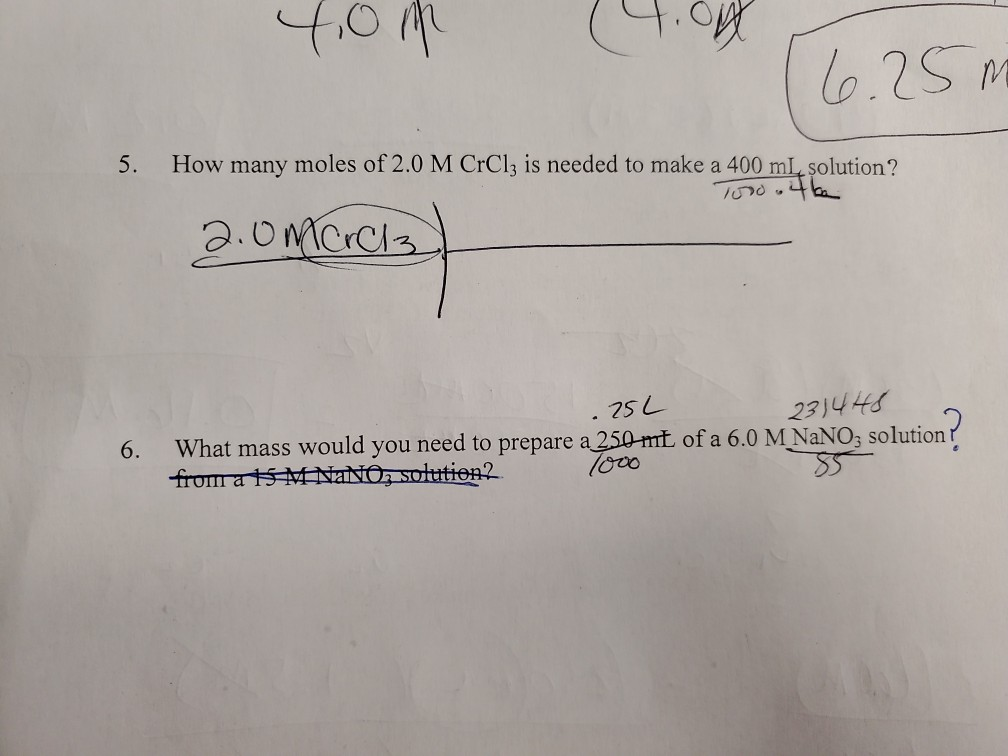16.25m 5. How many moles of 2.0 M CrClz is needed to make a 400 mL solution? Toro.tk 2.0mcrola .25L 6. 231448 What mass would you need to prepare a 250 mt of a 6.0 M NaNO3 solution from a H M NANO, solution?

• ### You need to prepare 100.0 mL of a pH=4.00 buffer solution using 0.100 M benzoic acid...

You need to prepare 100.0 mL of a pH=4.00 buffer solution using 0.100 M benzoic acid (pKa = 4.20) and 0.140 M sodium benzoate. How much of each solution should be mixed to prepare this buffer? mL benzoic acid = ? mL sodium benzoate = ?

• ### You want to make 500 mL of 10 M NaOH solutions? How much NaOH do you...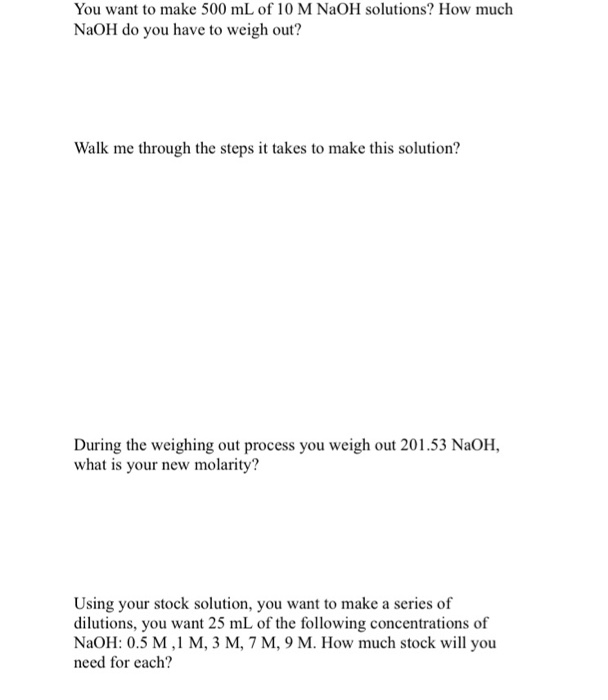You want to make 500 mL of 10 M NaOH solutions? How much NaOH do you have to weigh out? Walk me through the steps it takes to make this solution? During the weighing out process you weigh out 201.53 NaOH, what is your new molarity? Using your stock solution, you want to make a series of dilutions, you want 25 mL of the following concentrations of NaOH: 0.5 M ,1 M, 3 M, 7 M, 9 M. How much...